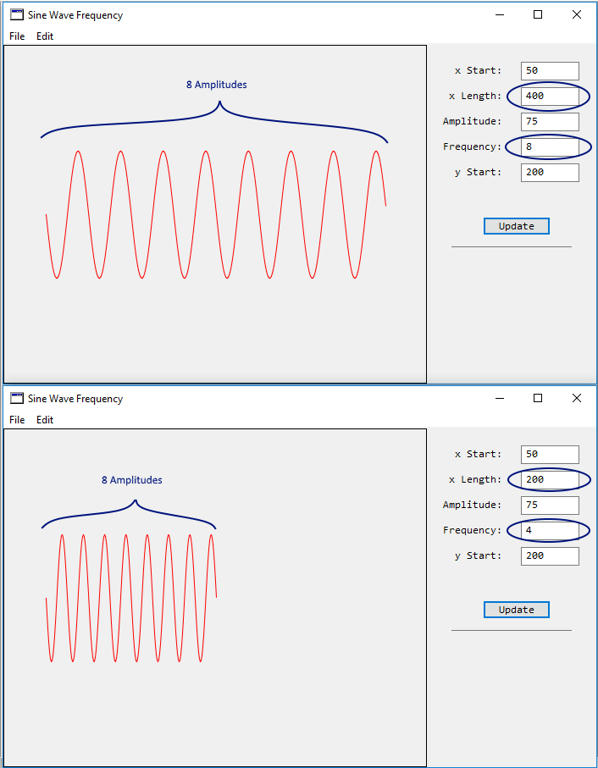Waves are difficult enough to generate, and how do you generate a wave that can be scaled? By scaling I mean that there is a constant number of amplitudes with a varying x-axis distance. This uses some of the code from the previous example at Make a Sine Wave. Scaling of a wave revolves around a modification of the code where the period is equal to the inverse of the frequency (period = 1/frequency), which has been changed in the program.A modified method for drawing the Wavy Line in Xojo code is shown below:

 Public Sub DrawWavyLine1(g as Graphics, XStart as Double, XLength as double, Amp as double, Frequency as Double, YStart as Double) //y = asin(b(x-h))+k //a = amplitude, b = period, h = horizontal shift, k = vertical shift, x = x-axis //XStart=Starting pixel coordinate on x-axis //XLength=Total length of the wave in pixels //Amplitude=Height of the wave from the middle to top of the wave in pixels //Frequency=Length of the repeating wave(Cycles per length unit) //YStart=Starting pixel coordinate on the y-axis Dim i as integer For i = 0 to XLength g.ForeColor = RGB(255,0,0) //Red g.DrawLine(i+XStart, Amp*sin((1/Frequency)*(i))+YStart, i+1+XStart, Amp*sin((1/Frequency)*(i+1))+YStart) Next i //Draw a border g.ForeColor = RGB(0,0,0) //Black g.DrawRect(0,0,g.Width, g.Height)End Sub

The way to have the same number of amplitudes for a given length is to change both the x-length and frequency by the same ‘factor’ for scaling. If the size of the wave is to be doubled by multiplying by 2, then multiply both the y-length value (from 200*2 = 400) and the Frequency (4*2=8), which will increase the size of the wave length and space the waves evenly.